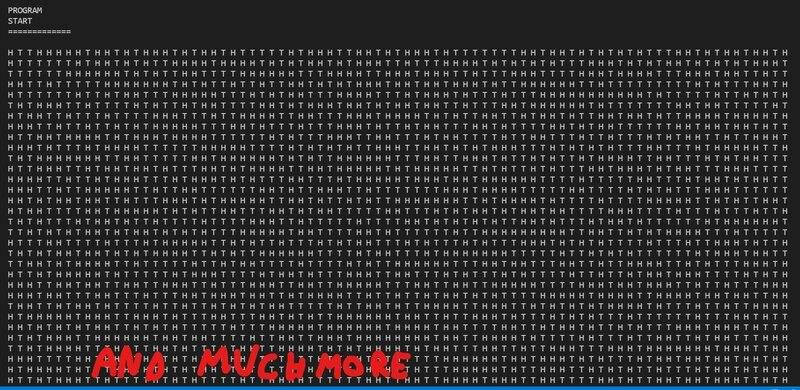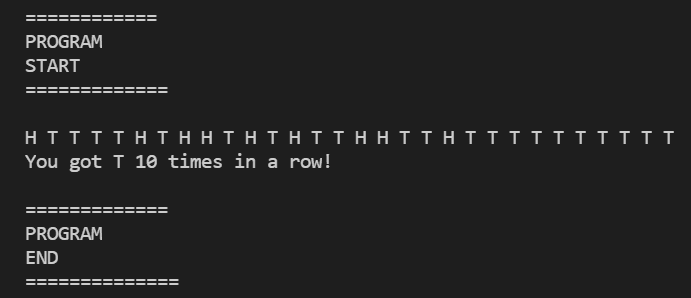Probability of 10 consecutive tails with 30 coin flips

• I
Summary:
How low is the probability of getting 10 consecutive tails with 30 coin flips?
Hi, i was doing a programming exercise that asked me to simulate te flip of coins until it finds 10 consecutive tails.
The program usually needs to flips like 6000/8000 coins before finding 10 tails consecutively, but suddenly i found 10 tails with only 30 coin flips, i think that what happened to me has a really low probaility.
I would like to know how low that probability is, can anyone help?

Usual output:Lucky outputphinds
Gold Member
2021 Award
Have you studied probability at all? What is your education level?

DaveC426913
Gold Member
Another line of approach is to not take for granted that your randomizer algorithm is random.

PeroK
Homework Helper
Gold Member
2021 Award
The odds of getting ten consecutive tails is ##\frac 1 {1024}##.

If we model this as a game where we restart every time a head comes up, then each game lasts two tosses on average. You can calculate this by analysing the binomial distribution.

You should, therefore, get ten tails in a row every ##2048## tosses. It's interesting that your program takes 6000+ tosses on average. Are you sure about that?

You should be able to simulate the game in a program to see how often you get ten tails inside thirty coin tosses.

It might not be to difficult to calculate the probability exactly. With a bit of thought.

•Fobi and Ibix
PeroK
Homework Helper
Gold Member
2021 Award
The probability of getting ten consecutive tails in thirty tosses or fewer is about ##\frac 1 {100}##.

Try writing a program to check that out.

Last edited:
•Ibix
PeroK
Homework Helper
Gold Member
2021 Award
PS I revised my estimate above.

Ibix
A little over 1% of sequences of 30 heads/tails contain a sequence of ten or more consecutive tails according to my testing.

After 100k tests, I find I need on average 2023 throws to get 10 consecutive tails. Not sure if that's disagreeing with @PeroK's 2048 or just the way the chances fell this time.

Certainly 6-8k throws on average looks high.

•Fobi and PeroK
Dale
Mentor
2021 Award
I also get about p=0.01 with Monte Carlo, but my calculation is off by a factor of 2. I figure that the probability of getting 10 tails is ##0.5^{10}## and there are 20 possible ways that can be done in 30 flips. But that gives p=0.02. So I am off by a factor of 2. It seems like the probability is ##0.5^9## but I don't see why.

Ibix
I don't think ##20\times 0.5^{10}## is correct. Consider strings of two or more heads in three throws. By explicit enumeration the answer is 3/8 (from eight combinations, TTH, HTT, and TTT contain two consecutive Ts), whereas your argument suggests 1/2. I think you are implicitly counting TTT twice, since it contains TT in both the first and second positions. I don't immediately see how that generalises to strings of ten out of thirty - but @PeroK originally wrote 1/50 before revising it to 1/100, so presumably he does.

(Incidentally, I tested my code on this case and it came up with ##0.3751\approx 3/8##, so I'm reasonably confident it's working. @Fobi might like to do the same, since it's easy to see if your code is working correctly with such short strings)

••Dale and PeroK
PeroK
Homework Helper
Gold Member
2021 Award
I also get about p=0.01 with Monte Carlo, but my calculation is off by a factor of 2. I figure that the probability of getting 10 tails is ##0.5^{10}## and there are 20 possible ways that can be done in 30 flips. But that gives p=0.02. So I am off by a factor of 2. It seems like the probability is ##0.5^9## but I don't see why.
You only get a shot at 10 tails from the 2nd toss onwards if the previous toss was a head. Otherwise you're double counting.

And @Ibix is right. That was my original mistake too.

•Dale and Ibix
Ibix
You only get a shot at 10 tails from the 2nd toss onwards if the previous toss was a head. Otherwise you're double counting.
Of course. Neat.

Dale
Mentor
2021 Award
Yes, that sounds right.

I tested the code again a few time and yes the average is lower than 6000/8000, here are some results
Number of flips: 1239
Number of flips: 1684
Number of flips: 521
Number of flips: 4854
Number of flips: 3561
Number of flips: 238
Number of flips: 4917
I am now making a function to get an average of like 1000 games to get a better approximation of the average flips

•PeroK
The average of 1000 is 2784

A little over 1% of sequences of 30 heads/tails contain a sequence of ten or more consecutive tails according to my testing.

After 100k tests, I find I need on average 2023 throws to get 10 consecutive tails. Not sure if that's disagreeing with @PeroK's 2048 or just the way the chances fell this time.

Certainly 6-8k throws on average looks high.
Yes i got a similar result running 30 flips game 100.000 times, i got that 1.252% have a 10 tails sequence, i thought that it would have been a much lower probability

FactChecker
Gold Member
Remember that when something that you think is rare happens, your question should be how likely is it that something that rare or rarer would happen. That is why @PeroK's change in post #5 is important.

•PeroK
Office_Shredder
Staff Emeritus
Gold Member
2021 Award
Fobi, a nice way to decide if your simulation is averaging enough trials is to just run it again, and see if you get a similar number

Ibix
You only get a shot at 10 tails from the 2nd toss onwards if the previous toss was a head. Otherwise you're double counting.
This isn't quite right, on further thought, because strings containing TTTTTTTTTTHTTTTTTTTTT satisfy your head-before-the-tails rule, but you're double counting still.

Here's an argument:

Consider throwing ten coins. There's exactly one sequence containing ten tails.
Now consider throwing another coin. The one sequence from ten throws becomes two (we don't care what the new coin is), and the one sequence that is one head followed by nine tails can become ten tails with the extra coin, for a total of three sequences containing ten tails.
Now consider throwing another coin. The three sequences become six, and there are additionally two sequences ending in nine tails that become ten tails, for a total of eight sequences containing ten tails.

In fact, in general, throwing another coin takes the existing sequences that include ten tails and doubles them, and adds ##2^{n-11}## new sequences, where ##n## is the total number of coins we've thrown (we need HTTTTTTTTTT at the end of the sequence, which is eleven throws, but any sequences are valid for the ##n-11## throws before that). You can work out that that is ##2^{n-10}+(n-10)2^{n-11}## sequences. But this pattern only holds up to ##n=20## (6,144 out of 1,048,576 sequences), because at ##n=21## we start to get sequences like my first paragraph.

Nevertheless, similar reasoning holds. Adding another coin takes our number of existing sequences and doubles it, and takes sequences that end in nine tails and makes them ten tails. You just need to remove the sequences that already have a run of ten in the first ##n-11## throws - and we know how many of those there are from above. If you track through all the doubling (which I did by mucking around in a spreadsheet), it comes down to ##2^{n-10}+(n-10)2^{n-11}-(n-17)\left(2^{n-22}+(n-22)2^{n-23}\right)##. That pattern holds to ##n=30##, which is fine for this problem.

So the exact answer is that with 30 coin tosses there are 11,517,696 sequences out of 1,073,741,824 possible sequences which have at least one run of at least ten tails. That's about 1.073%, which is more or less exactly what my Monte Carlo sim was saying.

I've actually tested this by explicit enumeration - the two formulae above match the results I get out to 30 throws.

@Fobi - 1.252% seems a bit high, so I suspect there's something still a bit off in your code.

•PeroK
PeroK
Homework Helper
Gold Member
2021 Award
This isn't quite right, on further thought, because strings containing TTTTTTTTTTHTTTTTTTTTT satisfy your head-before-the-tails rule, but you're double counting still.
The chance of getting two sets of ten tails in 30 tosses was small enough to ignore. I was only looking for a decent estimate.

In fact, ##\dfrac {11}{1024} \approx 0.01074## is my first approximation.

The probability of getting two sets of ten tails is small compared to that.

Last edited:
•mfb and Ibix
PeroK
Homework Helper
Gold Member
2021 Award
PS the probability adjustment to take account of the double counting of two sequences of ten tails would be.

Note that apart from the first ten coins, I demanded that we have a Head followed by 10 tails. That means we have 45 cases of double counting:

1-10 and 12-21 or 13-22 ... 21-30
2-11 and 13-22 ...
...
10-19 and 21-30

Apart from the first line the probability of each is ##(1/2048)^2##. The first line (10 cases) has twice that probability.

In any case, the adjustment is ##-\dfrac{65}{2048^2}##.

This gives a precise answer of ##0.0107266903##.

Which is the same as @Ibix got.

PeroK
PS to summarise$$p = \frac {11}{1024} - \frac {65}{2048^2}$$